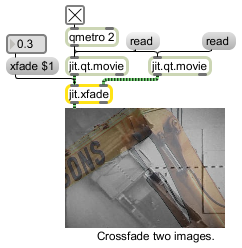## Description

The jit.xfade object crossfades between two matrices. A crossfade value of 0 results in output values equivalent to the left input values, while a crossfade value of 1 results in output values equivalent to the right input matrix.

## Matrix Operator

matrix inputs:2, matrix outputs:1
 Name IOProc Planelink Typelink Dimlink Plane Dim Type in2 resamp 1 1 1 1 1 char long float32 float64 out n/a 1 1 1 1 1 char long float32 float64

## Attributes

Name Type g/s Description
xfade float The amount of crossfade between the two input matrices (default = 0.) A value of 0. outputs only the matrix received in the left input, and a value of 1. outputs only the matrix received in the right input.

## Examples